Reach Us+44-1474-556909
Reflecting About Selecting Noninformative Priors | OMICS International
Journal of Applied & Computational Mathematics
All submissions of the EM system will be redirected to Online Manuscript Submission System. Authors are requested to submit articles directly to Online Manuscript Submission System of respective journal.

Kamary K1* and Robert CP2

1CEREMADE, Universite Paris-Dauphine, 75775 Paris cedex 16, France

2CREST-Insee and CEREMADE, Universite Paris-Dauphine, 75775 Paris cedex 16, France

*Corresponding Author:
Kaniav Kamary
75775 Paris cedex 16, France
Tel: 33144054405
E-mail:[email protected]

Received July 04, 2014; Accepted July 18, 2014; Published July 21, 2014

Citation: Kamary K, Robert CP (2014) Reflecting About Selecting Noninformative Priors. J Appl Computat Math 3: 175. doi: 10.4172/2168-9679.1000175

Copyright: © 2014 Kamary K, et al. This is an open-access article distributed under the terms of the Creative Commons Attribution License, which permits unrestricted use, distribution, and reproduction in any medium, provided the original author and source are credited.

Visit for more related articles at Journal of Applied & Computational Mathematics

Abstract

Following the critical review of Seaman et al., we react on an essential aspect of Bayesian statistics, namely the selection of a prior density. In some cases, Bayesian data analysis remains stable under different choices of noninformative prior distributions. However, as discussed by Seaman et al., there may also be unintended consequences of a choice of noninformative prior and, according to these authors, this is a problem often ignored in applications of Bayesian inference". They focused on four examples, analyzing each for several choices of prior. Here, we reassess these examples and their Bayesian processing via different prior choices for fixed data sets. The conclusion is to infer the overall stability of the posterior distributions and to consider that the effect of reasonable noninformative priors is mostly negligible.

Keywords

Induced prior; Logistic model; Bayesian methods; Stability; Prior distribution

Introduction

The choice of a particular prior for a model can be thought as a science in itself or even as an art. When we want to use Bayesian modeling but fail to gather useful information about the prior distribution, the solution is to resort to some statistical distributions named noninformative priors. This choice may be purely mathematical and used as such, even though the posterior distribution is proper and hence a correct density function, it is nonetheless open to criticism. In particular, and this will be the focus of this note, Seaman et al.  claimed that using a particular noninformative distribution is a problem in itself, often ignored by users of these priors. The argument goes as follows: if parameters with diffuse proper priors are subsequently transformed, the resulting induced priors can, of course, be far from diffuse, possibly resulting in unintended influence on the posterior of the transformed parameters". Also applications typically employ Markov chain Monte Carlo (MCMC) methods to obtain posterior features, resulting in the need for proper priors, even when the modeler prefers that priors be relatively noninformative", which confuses proper priors with proper posteriors and is used to restrict the focus solely (and inappropriately in our opinion) on proper priors.

More precisely, Seaman et al.  investigated side effects of some particular prior choices through examples. This note aims at reexamining this investigation and giving a brief discussion on these topics in the following sections. First, note that a prior is considered as informative by Seaman et al.  to the degree it renders some values of the quantity of interest more likely than others", and with this definition, when comparing two priors, the prior more informative is deemed preferable. In contrast to this definition, we stress that an informative prior expresses specific, definite information about the parameter, providing quantitative numerical information that is crucial to the estimation of a model. As pointed out by Robert , if there is information about the parameters, the prior distributions need to include this information in. However in most practical cases, the parameter has no reality of its own but rather corresponds to a parameterization of the law describing the random phenomenon observed therein. The prior is a tool employed to summarize the information available on this phenomenon, as well as the uncertainty within the Bayesian structure. There are many discussions of how insight and guidance into appropriate choices between the prior distributions might be obtained. In this case, robustness considerations also have an interesting role to play [3,4]. This point of view will be obvious in this paper through our Bayesian processing a logistic model for three different noninformative priors. Bayesian robustness modeling distributions provide a flexible approach to resolving problems and conflicts between the data and prior distributions . Also, we can model uncertainty in the prior by specifying a class of possible prior distributions to the parameters [6,7]. For the examples processed in Seaman et al. , we exhibit stability in the posterior distributions through various noninformative priors. We first provide a brief review of noninformative priors in Section 2. In Section 3, we will thus run a Bayesian analysis on a logistic model  by choosing the normal distribution N (0,σ2) as the regression coefficient prior. We then compare it with a g-prior, as well as at and Jeffreys' priors, concluding to the stability of our results. The next sections cover the second to fourth examples of Seaman et al. , modeling covariance matrices, treatment effect in biomedical studies, and a multinomial distribution index. When modeling covariance matrices, we compare two default priors for the standard deviations of the model coefficients. In the multinomial setting, we discuss the hyperparameters of a Dirichlet prior. Finally, we conclude with the argument that the use of noninformative priors is reasonable within a fair range and that they provide efficient Bayesian estimations when the information about the parameter is vague or very poor.

Noninformative Priors

As mentioned above, if prior information is not available and if we stick to Bayesian modeling, we need to resort to the so-called noninformative priors. Since we want a prior with minimal impact on the final inference, we define a noninformative prior as a statistical distribution that expresses vague or general information about the parameter in which we are interested. In constructive terms, historically, the first rule for determining a noninformative prior is the principle of indifference, using uniform distributions which assign equal probabilities to all possibilities . This distribution however is not invariant under reparametrization and invariant non informative priors were later defined [2,9]. If the problem does not have an invariance structure, Jeffreys' priors, then reference priors, exploit the structure of the problem under study in a more formalized way. Other methods are available, like the little-known data-translated likelihood of Box et al. , maxent priors and probability matching priors . Bernardo et al.  regard the noninformative prior as a mathematical tool and that these priors are introduced as a category of priors that minimize the impact of the prior selection on inference: Put bluntly, data cannot ever speak entirely for themselves, every prior specification has some informative posterior or predictive implications and vague is itself much too vague an idea to be useful. There is no\objective" prior that represents ignorance". It is obvious that prior distributions can never be quantified or elicited exactly, especially when there is no information on those parameters. So, the concept of true prior is meaningless and quantification of prior beliefs is done with uncertainty. As Berger et al.  has noted, noninformative priors have the advantage that they can be considered to provide robust solutions to problems and the user of these priors should be concerned with robustness with respect to the class of reasonable noninformative priors".

Example 1: Bayesian Analysis of the Logistic Model

The first example in Seaman et al.  is a simple logistic regression with probability of coronary heart disease depending on the age x by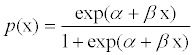(1)

First we review the original analysis of Seaman et al.  and then run our own analyze by selecting normal distribution as well as the g-prior, the flat prior and Jeffreys' prior.

The original analysis

For both parameters of the model (1), Seaman et al.  chose a normal prior N (0,σ2). The first surprising feature in this choice is to see an identical prior on both intercept and slope coefficients, instead of, e.g., a g-prior (discussed in the following) that would rescale the coefficients according to the variation of the corresponding covariate. Since x corresponds to age, the second term βx in the regression varies 50 times more when compared with the intercept. When plotting the resulting logistic cdf across a few thousands simulations from the prior, the cumulative functions mostly end up as constant functions with values 0 or 1. This is obviously not particularly realistic since the predicted phenomenon is the occurrence of coronary heart disease. The prior is thus using the wrong scale: the simulated cdfs should have a reasonable behavior over the range (20,100) of the covariate x. For instance, it should be focusing on a -5 log-odds ratio at age 20 and a+5 log-odds ratio at 100, leading to the comparison pictured in Figure 1 (left versus right). Furthermore, the fact that the coefficient of x may be negative is also ignoring a basic issue about the model and answers the later self-criticism in Seaman et al.  that the prior probability that the ED50 is negative is 0:5. Using instead a flat prior here would answer the authors' criticisms about the prior behavior, as we now demonstrate. We stress that Seaman et al.  advance no explanation for the choice of the prior variance σ2=252, other than there is no information about the model parameters. This is a completely arbitrary choice of prior, which does have a considerable impact on the inference that follows, as shown in Figure 1 (left). Seaman et al.  further criticized the chosen prior by comparing both posterior mode and posterior mean derived from the normal prior assumption with the MLE. If the MLE is the golden standard there then one may wonder about the reference of a Bayesian analysis! We recall that, when the sample size N gets large, many simple Bayesian analyses based on noninformative prior distributions give results similar to standard non-Bayesian approaches . From a Bayesian data analysis perspective, we can often interpret classical point estimates as exact or approximate posterior summaries based on some implicit full probability model. For example, Lopes et al.  have shown that a Bayesian posterior mean under a conjugate prior and the frequentist MLE are asymptotically equivalent for exponential families. Therefore, as the sample size increases, the influence of the prior on posterior inferences decreases and when N tends to infinity, most priors lead to exactly the same inference. However, for smaller sample sizes, it is inappropriate to summarize inference about the parameter by one value like the mode or the mean, especially when the posterior distribution of the parameter is more variable or even asymmetric. The data set used here to infer on (α,β) is the Swiss banknote benchmark (available in the R language). The response variable y indicates the states of the banknote, whether the banks note is genuine or counterfeit. The explanatory variable is the bill length. This data yields the maximum likelihood estimations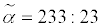and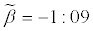. To check the impact of the normal prior variance, we used a random walk Metropolis-Hastings algorithm as in Marin et al.  and derived the estimators reproduced in Table 1. We can spot definitive changes in the results that are caused by changes in the coefficient σ, hence concluding to the clear sensitivity of the posterior to the choice of hyperparameter σ (Figure 2).

Figure 1: Logistic cdfs across a few thousands simulations from the normal prior, when using the prior selected by Seaman et al.  (left) and the prior defined as the G-prior (right).

Figure 2: Posterior distributions of α when priors are N (0, σ) for σ=10, 25, 100, 900, based on 104 MCMC simulations.

 σ=10 α β mean s.d mean s.d 3.482 11.6554 -0.0161 0.0541 σ= 25 18.969 24.119 -0.0882 0.1127 σ=100 137.63 64.87 -0.6404 0.3019 σ=900 237.2 86.12 -1.106 0.401

Table 1: Posterior estimates using a normal prior when σ=10, 25, 100, 900.

Larger classes of priors

Picking normal priors being far from robust , we can limit variations in the posteriors, using the g-priors of ,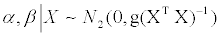(2)

where the prior variance-covariane matrix is a scalar multiple of the information matrix for the linear regression. This coefficient g plays a decisive role in the analysis, however large values of g imply a more diffuse prior and, as shown e.g. in Marin et al.  if the value of g is large enough, the Bayes estimate stabilizes. We will select g as equal to the sample size 200, following Liang et al.  as it means that the amount of information about the parameter is equal to the amount of information contained in one single observation. Our second proposed prior is the flat prior π (α, β)=1. And Jeffreys' prior constitutes our third prior as in Marin et al. . In the logistic case, Fisher's information matrix is

I (α, β, X)=XTWX,

where X={xir} is the design matrix, W=diag {mi πi (1- πi)} and mi is the binomial index for the ith count . This leads to Jeffreys' prior {det(I α, β, X))}1/2 proportional to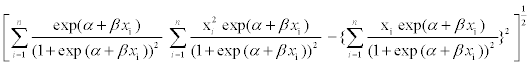(3)

This is a nonstandard distribution on (α, β) but it can easily approximated by a Metropolis-Hastings algorithm whose proposal is the normal Fisher approximation of the likelihood, as in Marin et al. . All point estimates in Table 2 are averages of posterior samples of 104 simulations.

g-prior
α β
mean s.d mean s.d
237.63 88.0377 -1.1058 0.4097
Flat prior
236.44 I 85.1049 I -1.1003 I 0.3960
Jeffreys' prior
237.24 87.0597 -1.104 0.4051

Table 2: Posterior estimates under a g-prior, a flat prior and Jeffreys' prior for the banknote benchmark. Posterior means and standard deviations remain quite similar under all priors.

Range of estimates

Bayesian estimates of the regression coefficients associated with the three noninformative priors above are summarized in Table 2. Those estimates vary quite moderately from one choice to the next, as well as relatively to the MLEs and to the results shown in Table 1 when σ=900. Figure 3 is even more definitive about this. There is no significant difference between those and we conclude at the stability of Bayesian inferences under these different prior choices.

Figure 3: Posterior distributions of the parameters of the logistic model when the prior is N (0, 9002), g-prior, at prior and Jeffreys' prior, respectively. The estimated posterior distributions are based on 104 MCMC iterations.

Example 2: Modeling Covariance Matrices

The second choice of prior criticized by Seaman et al. , was proposed by Barnard et al.  for the modeling of covariance matrices. However the paper falls short of demonstrating a clear impact of this prior modeling on posterior inference. Furthermore the solution of using another proper prior resulting in a \wider" dispersion requires a prior knowledge of how wide is wide enough. We thus assess here the evaluated regression model and then run Bayesian analyses considering both prior beliefs specified by Seaman et al. and Barnard et al. [1,18].

Setting

The multivariate regression model of interest is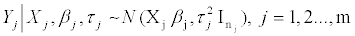(4)

where Yj is a vector of nj dependent variables, Xj is an nj×k matrix of covariate variables, and βj is a k-dimensional parameter vector. For this model, Barnard et al.  considered an iid normal distribution as the prior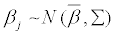conditional on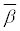, Σ where,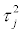for j=1, 2, …, m are independent and follow a normal and inverse-gamma priors, respectively. Assuming that, andare a priori independent. Barnard et al.  firstly provide a full discussion on how to choose a prior for Σ because the nature of the shrinkage of the posterior of the individual βj is determined by it towards a common target. The covariance matrix Σ is defined as a diagonal matrix with diagonal elements S, multiplied by a k×k correlation matrix R, “Σ=diag (S) R diag (S)". Note that S is the k×1 vector of standard deviations of βjs, (S1, …, Sk). Barnard et al.  propose lognormal distributions as priors on Sj and while the correlation matrix could have (1) a joint uniform prior which means p (R) α 1, or (2) a marginal prior obtained from the inverse-Wishart distribution for Σ which means p (R) is derived from the integral over S1,…, Sk of a standard inverse-Wishart distribution. In the second case, all the marginal densities for rij are uniform when i≠j, (see Barnard et al. . Seaman et al.  chose a different prior structure, with a prior on the correlations and a lognormal prior with means 1, -1 and standard deviations 1, 0.5 on the standard deviations of the intercept and slope, respectively. Simulating from this prior, they concluded at a high concentration near zero. They then concluded that the lognormal distribution should be replaced by a gamma distribution G (4, 1) as it implied a more diffuse prior. The main question here is whether or not the induced prior is more diffuse should make us prefer gamma to lognormal as a prior for Sj, as discussed below.

Prior beliefs

First, Barnard et al.  basic modeling intuition is “that each regression is a particular instance of the same type of relationship". This means an exchangeability prior belief on the regression parameters. As an example, they suppose that m regressions are similar models where each regression corresponds to a different firm in the same industry. Exploiting this assumption, when βj has a normal prior like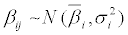, j=1, 2,…,m, the standard deviation of βij (Sii ) should be small as well so\that the coefficient for the ith explanatory variable is similar in the different regressions". In other words, Si concentrated on small values implies little variation in the ith coefficient. Toward this goal, Barnard et al.  chose a prior concentrated close to zero for the standard deviation of the slope so that the posterior of this coefficient would be shrunken together across the regressions. Based on this basic idea and taking tight priors on Σ for βj, j=1,…, m, they investigated the shrinkage of the posterior on βj as well as the degree of similarity of the slopes. Their analysis showed that a standard deviation prior that is more concentrated on small values results in substantial shrinkage in the coefficients relative to other prior choices. Consider for instance the variation between the choices of lognormal and gamma distributions as prior of S2, standard deviation of the regression slopes. Figure 4 compares the lognormal prior with normal mean and standard deviation -1, 0.5 and the gamma distribution G (4, 1). In this case, most of the mass of the lognormal prior is concentrated on values close to zero whereas the gamma prior is more diffuse. The 10, 50, 90 percentiles of LN (-1, 0.5) and G (4, 1) are 0.19, 0.37, 0.7 and 1.74, 3.67, 6.68, respectively. Thus, choosing LN (-1, 0.5) as the prior of S2 is equivalent to believe that values of β2 in the m regressions are much closer together than the situation where we assume S2 ~ G (4, 1). To assess the difference between these two prior choices on S2 and their impact on the degree of similarity of the regression coefficients, we resort to a simulated example. In short, our example is similar to that defined in Barnard et al. , except for different values of k=1, number of the regression coefficients, m=4, number of normal regressions, and nj=36, number of observations. The explanatory variables are simulated from the standard normal distribution. We also take τj ~ I G (3, 1) and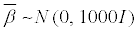. The prior for Σ is such that π (R) α 1 and we run Seaman et al.'s  analyses under S2 ~ LN (-1, 0.5) and S2 ~ G (4, 1).

Comparison of posterior outputs

Using 104 Gibbs sampling simulations, we produce the estimates and standard deviations of Tables 3 and 4, respectively. The difference between the regression estimates is quite limited from one prior to the next, while the estimates of the standard deviations vary much more. In the lognormal case, the posterior of Si is concentrated on smaller values relative to the gamma prior. Figure 5 displays the posterior estimations of the regression intercept, slope and Si, i=1, 2 simulated from a Gibbs sampler based on 104 iterations. The impact of the prior choice is quite clear on the standard deviations. Since of intercepts and slopes for all four regressions are centered in (16, 5, 17) and (-10, -9), respectively, we can conclude at the stability of Bayesian inferences on βj when selecting two different prior distributions on Sj.

Si~ LN (-1, 0.5)
Regression 1 Regression 2 Regression 3 Regression 4
Estimate mean sd mean sd mean sd mean sd
Intercept 16.74 0.17 16.72 0.17 16.79 1.09 16.82 0.69
Slope -9.27 0.42 -9.47 0.25 -9.66 0.98 -9.63 0.45
Si ~ G (-4, 1)
Regression 1 Regression 2 Regression 3 Regression 4
Estimate mean sd mean sd mean sd mean sd
Intercept 16.73 0.23 16.73 0.22 16.85 0.37 16.76 0.32
Slope -9.3 0.3 -9.47 0.34 -9.73 0.23 -9.64 0.8

Table 3: Posterior estimations of regression coefficients when their standard deviations are distributed as LN (-1, 0.5) versus G (4, 1).

Si ~ LN (-1, 0.5)
Regression 1 Regression 2 Regression 3 Regression 4
Estimate mean sd mean sd mean sd mean sd
S1 0.43 0.27 0.44 0.26 0.42 0.26 0.41 0.24
S2 0.42 0.27 0.43 0.25 0.42 0.25 0.43 0.32
Si ~ G (-4, 1)
Regression 1 Regression 2 Regression 3 Regression 4
Estimate mean sd mean sd mean sd mean sd
S1 2.31 1.28 2.33 1.29 2.29 1.29 2.29 1.26
S2 2.32 1.29 2.23 1.28 2.25 1.23 2.3 1.26

Table 4: Posterior estimations standard deviations of the regression coefficients when their priors are distributed as LN (-1, 0.5) versus G (4, 1).

Figure 5: Estimated posterior densities of the regression intercept (top left), slope (top right), standard deviation of the intercept (down left) and standard deviation of the slope (down right), respectively for 4 different normal regressions. All estimates based on 104 iterations that were simulated from a Gibbs sampler.

Examples 3 and 4: Prior Choices for a Proportion and the Multinomial Coefficients

This section considers more briefly the third and fourth examples of Seaman et al. . The third example relates to a treatment effect analyzed by Cowles  and the fourth one covers a standard multinomial setting. 5.1 proportion of treatment effect captured In Cowles  two models are compared for surrogate endpoints, using a link function g that either includes the surrogate marker or not. The quantity of interest is a proportion of treatment effect captured which is defined as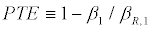where β1, βR,1 are the coefficients of an indicator variable for treatment in the first and second regression models, respectively. Seaman et al.  restricted this proportion to the interval (0, 1) and under this assumption they proposed to use a kind of beta distribution (conditional beta distribution) on β1, βR,1 so that PTE stayed within (0; 1).We find this example intriguing in that, even if PTE could be turned into a meaningful quantity (given that it depends on parameters from different models), the criticism that it may take values outside (0, 1) is rather dead-born since it suffices to impose a joint prior that ensures the ratio stays within (0, 1). This actually is the solution eventually proposed by the authors. If we have prior beliefs about the parameter space (which depends on β1, βR,1 in this example) the prior specified on the quantity of interest should integrate these beliefs. In the current, there is seemingly no prior information about (β1, βR,1) and hence imposing a prior restriction to (0; 1) is not a logical specification. For instance, using normal priors on β1, and βR,1 lead to a Cauchy prior on β1R,1, which support is not limited to (0, 1). We will not discuss this example any further.

Multinomial model and evenness index

The final example in Seaman et al.  and in this paper deals with a measure called evenness index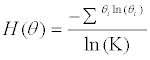that is a function of a vector θ of proportions θi, i=1, … , K. The authors assume that these are associated with a Dirichlet prior with parameters first equal to 1 then to 0.25. For the selection function H, the first prior concentrates on (0, 5, 1) whereas the second does not. Since there is nothing special about the uniform, re-running the evaluation with a Jeffreys prior reduces this feature, which anyway is a characteristic of the prior distribution, not of the posterior distribution which accounts for the data. The authors actually propose to use the Dir (1/4, 1/4,…,1/4) prior, presumably on the basis that the induced prior on the evenness is then centered close to 0.5.

If we consider the more generic Dir (Ɣ1,…,Ɣ K) prior, we can investigate the impact of the Ɣis when they move from 0:1 to 1. Discussion: We compare the values 0.1, 0.25, 0.5, 1 for the Ɣi’ s.. Figure 6 shows the corresponding priors on H(θ): the concentration of the density of the evenness index on (0.5, 1) decreases by reducing Ɣi. For Ɣi=0.1, it is concentrated on (0, 0.7) while for Ɣi=0.1 most of the mass is on values between 0 and 0.5. To further the comparison, we generated datasets each of sizes N=50, 100, 250, 1000, 10, 000. Figure 7 shows the posteriors associated with each of the four Dirichlet priors for these sample sizes, including their mode which are all close to 0.4 when N=104. Even for moderate sample sizes like 50, the induced posteriors are almost similar. The posterior means on H(θ), are reproduced in Table 5. When the sample size is 50, there is a substantial variation, however, between the posterior means such that the as the sample size increases this difference decreases to zero.

 Sample size 50 100 250 1000 10,000 Dirichlet prior when - Ɣi=0.1 Posterior mean 0.04 0.34 0.4 0.38 0.395 Dirichlet prior when - Ɣi=0.25 Posterior mean 0.32 0.44 0.42 0.39 0.396 Dirichlet prior when  Ɣi=0.5 Posterior mean 0.38 0.37 0.42 0.39 0.397 Dirichlet prior when Ɣi=1 Posterior mean 0.45 0.43 0.44 0.39 0.396 Jeffreys' prior: Ɣi=0.125 Posterior mean 0.41 0.41 0.41 0.39 0.396 Posterior s.d 0.06 0.06 0.04 0.02 0.006

Table 5: Posterior means of H(θ) for the priors shown in Figure 6 and Jeffreys' prior on θ for sample sizes 50, 100, 250, 1000, 10, 000.

Figure 6: Induced priors on the evenness index: Four Dirichlet prior is assigned to ϴ with hyperparameters all equal to 0.1, 0.25, 0.5, 1.

Figure 7: Estimated posterior densities of H(ϴ) considering sample sizes of 50, 100, 250, 1000, 10, 000. They correspond to the priors on ϴ shown in Figure 6 and are based on 104 posterior simulations. The vertical line indicates the mode of all posteriors when sample size is large enough.

Since the Dirichlet distributions are conjugate priors, hence possibly lacking in robustness, we propose to set Ɣi=1/K (here K is equal to 8) which transforms Dirichlet distribution to a Jeffreys' prior. This non informative prior works well and could minimize the influence of the prior input on the inferential output for small sample sizes . Figure 8 reproduces the transform of Jeffreys' prior for the evenness index (left) and the induced posterior densities for N=50, 100, 250, 1000, 10, 000 (right). Once again, the posteriors concentrate around 0.4 even though Jeffreys' prior is more diffuse than the other proposal priors of 6. The last two rows of Table 5 displays means and standard deviations of simulated posterior distributions on H(θ)for Jeffreys' prior. The same stability occurs.

Figure 8: Jeffreys' prior and estimated posterior densities of H(ϴ) considering sample sizes 50, 100, 250, 1000, 10, 000. The posterior distributions are based on 104 posterior draws. The vertical line indicates the mode of the posterior density when the sample size is 104.

Conclusion

In this note, we reassessed the examples of the critical review of Seaman et al. . Our own Bayesian modeling was based on non informative priors such as g-priors, at and Jeffreys' priors, as well as weakly informative priors . According to the outcomes produced therein, the use of non informative distributions as priors result in stable posterior inferences and also give reasonable Bayesian estimations for the parameters at hand. We thus consider the level of criticism found in the original paper rather superficial, as it either relies on a highly specific choice of a proper prior distribution or on ignoring basic prior information. The paper of Seaman et al.  concludes with recommendations for prior checks. The recommendations are mostly sensible if expressing the fact that some prior information is almost always available on some quantities of interest. Our only point of contention is the repeated and recommended reference to MLE, since it implies assessing or building the prior from the data. The most specific (if related to the above) recommendation is to use conditional mean priors as exposed by Christensen et al. . For instance, in the first (logistic) example, this meant putting a prior on the cdfs at age 40 and age 60. The authors picked a uniform in both cases, which sounds inconsistent with the presupposed shape of the probability function. In conclusion, we find there is nothing pathologically wrong with either the paper of Seaman et al.  or the use of “non informative" priors! Looking at induced priors on more intuitive transforms of the original parameters is a commendable suggestion, provided some intuition or prior information is already available on those. Using a collection of priors including reference or invariant priors helps as well to build a feeling about the appropriate choice or range of priors and looking at the induced dataset by simulating from the corresponding predictive cannot hurt.

References

Select your language of interest to view the total content in your interested language

Article Usage

• Total views: 12526
• [From(publication date):
September-2014 - Jun 26, 2019]
• Breakdown by view type
• HTML page views : 8725Can't read the image? click here to refresh